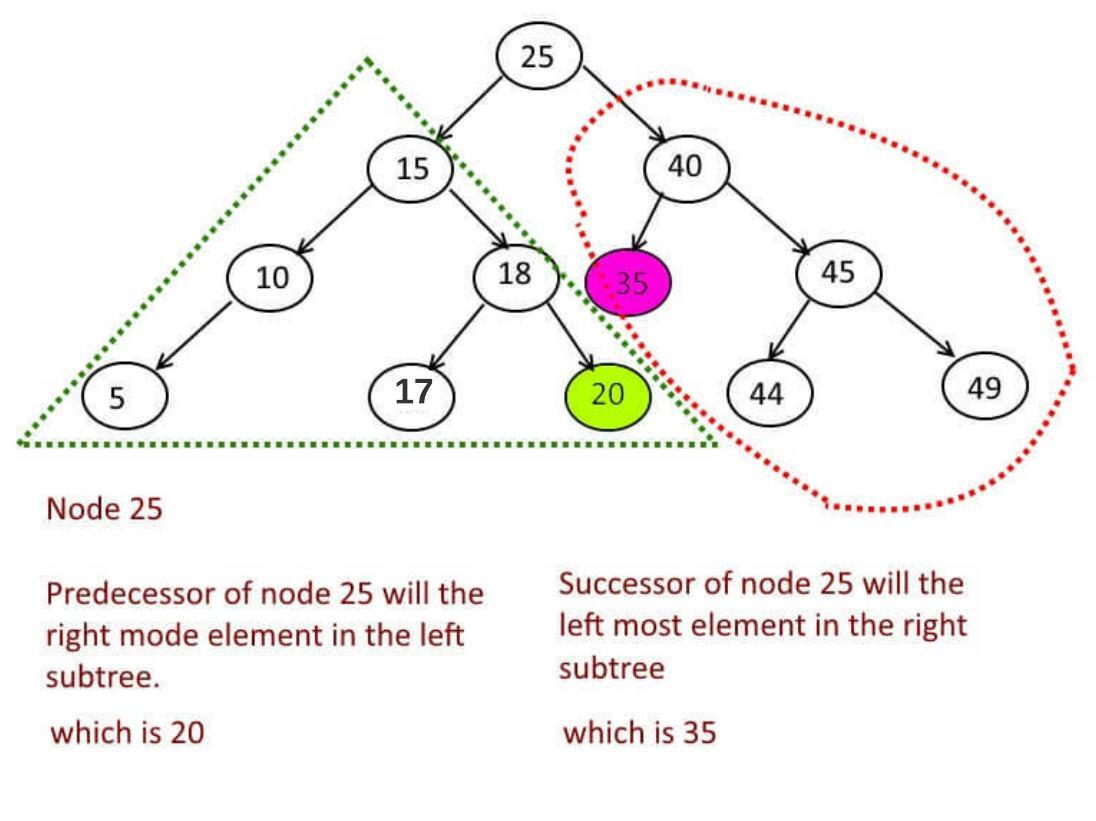Hard

# Inorder Predecessor and Successor in Binary Search Tree

Objective: - Given a Binary Search Tree, Find predecessor and Successor of a given node.

What is Predecessor and Successor :

When you do the inorder traversal of a binary tree, the neighbors of given node are called Predecessor(the node lies behind of given node) and Successor (the node lies ahead of given node).

Example:Approach:

Say you have to find the inorder predecessor and successor node 15.

• First compare the 15 with root (25 here).
• 25>15 => successor = 25, make recursive call to root.left.(Why do we do it , is explained at step 3).
• New root which is = 15, now stop making recursive calls.
• Now successor will be the left most node in right subtree of which has the root 18 here. So the left most element and successor will be 19. (What if 15 doesn't have a right subtree, then successor of 15 will be the parent of it, and that is why we set parent as successor in step1).
• Now predecessor will be the right most node in left subtree which has the root 10 here. but 10 doesn't have right child.
• For the same reason when node<root then make predecessor = root and make a recursive call to the root.right.

Code:

Output:

```Inorder Successor of 10 is : 13 and predecessor is : 7
Inorder Successor of 30 is : 35 and predecessor is : 25
```